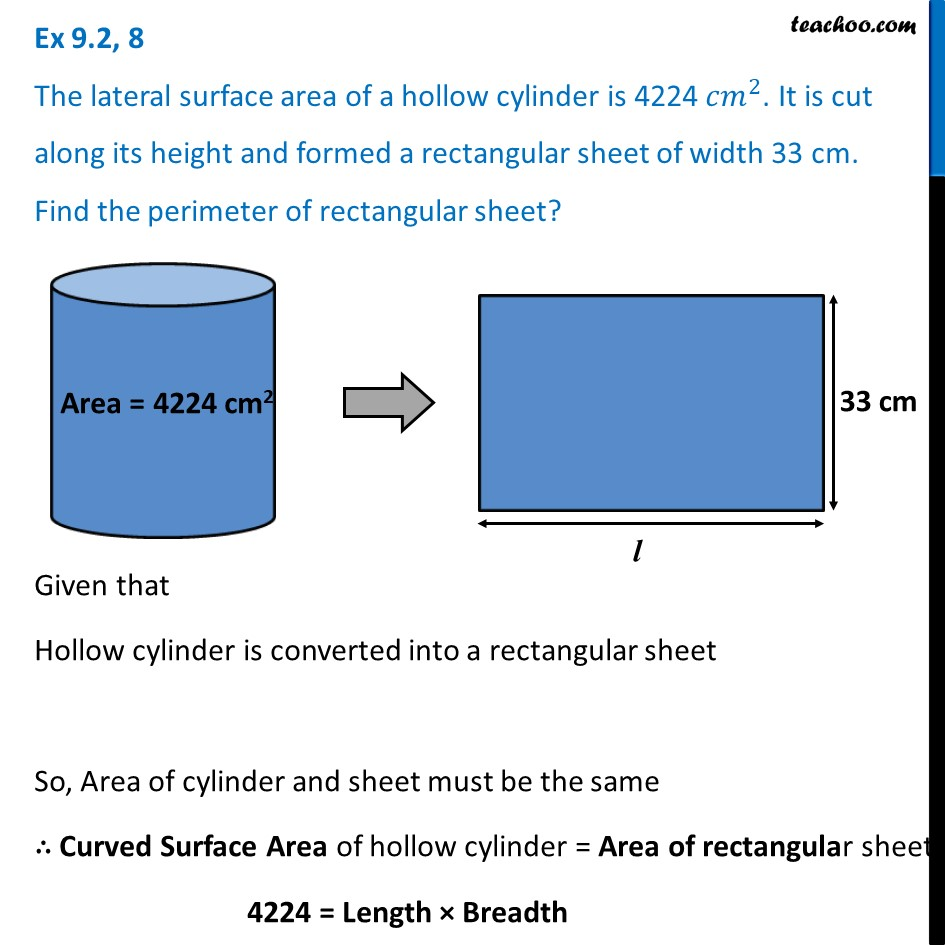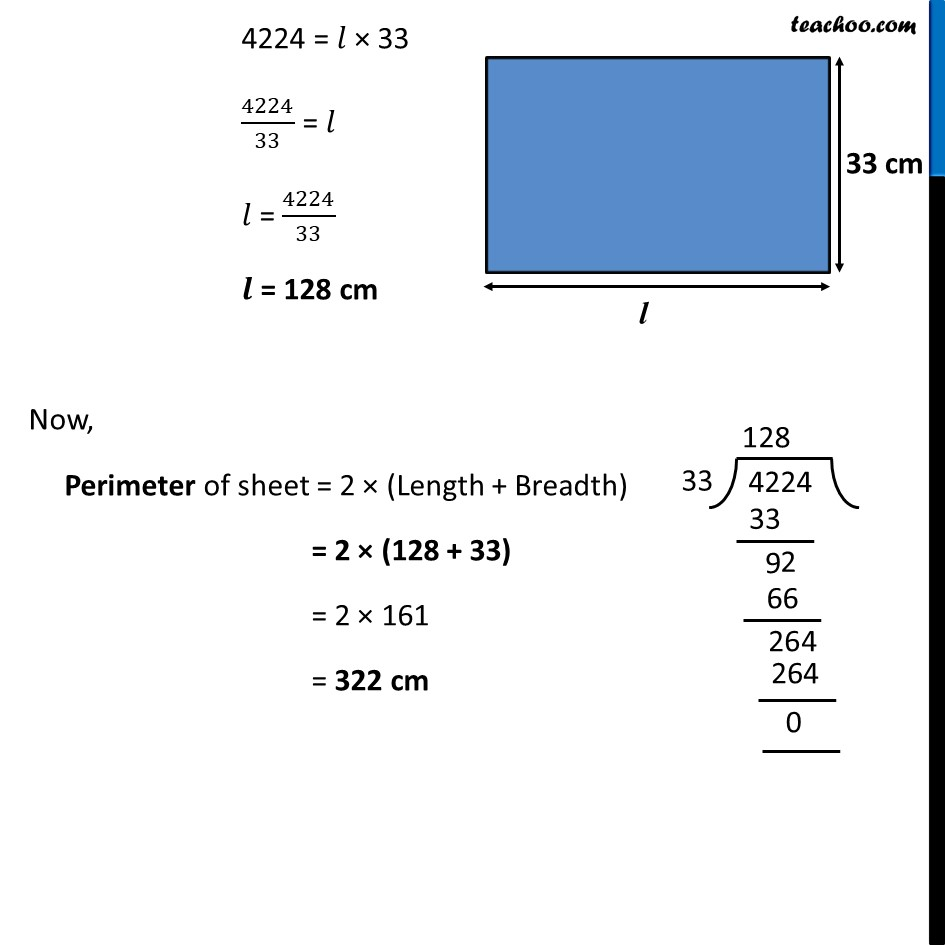Ex 9.2

Chapter 9 Class 8 Mensuration
Serial order wiseLearn in your speed, with individual attention - Teachoo Maths 1-on-1 Class

### Transcript

Ex 9.2, 8 The lateral surface area of a hollow cylinder is 4224〖 𝑐𝑚〗^2. It is cut along its height and formed a rectangular sheet of width 33 cm. Find the perimeter of rectangular sheet?Given that Hollow cylinder is converted into a rectangular sheet So, Area of cylinder and sheet must be the same ∴ Curved Surface Area of hollow cylinder = Area of rectangular sheet 4224 = Length × Breadth 4224 = 𝑙 × 33 4224/33 = 𝑙 𝑙 = 4224/33 𝒍 = 128 cm Now, Perimeter of sheet = 2 × (Length + Breadth) = 2 × (128 + 33) = 2 × 161 = 322 cm